# Decision Tree Regression With Hyper Parameter Tuning

In this post, we will go through Decision Tree model building. We will use air quality data. Here is the link to data.

In :
import pandas as pd
import numpy as np

In :
# Reading our csv data

Out:
Unnamed: 0 T TM Tm SLP H VV V VM PM 2.5
0 1 26.7 33.0 20.0 1012.4 60.0 5.1 4.4 13.0 284.795833
1 3 29.1 35.0 20.5 1011.9 49.0 5.8 5.2 14.8 219.720833
2 5 28.4 36.0 21.0 1011.3 46.0 5.3 5.7 11.1 182.187500
3 7 25.9 32.0 20.0 1011.8 56.0 6.1 6.9 11.1 154.037500
4 9 24.8 31.1 20.6 1013.6 58.0 4.8 8.3 11.1 223.208333

T == Average Temperature (°C)

TM == Maximum temperature (°C)

Tm == Minimum temperature (°C)

SLP == Atmospheric pressure at sea level (hPa)

H == Average relative humidity (%)

VV == Average visibility (Km)

V == Average wind speed (Km/h)

VM == Maximum sustained wind speed (Km/h)

PM2.5== Fine particulate matter (PM2.5) is an air pollutant that is a concern for people's health when levels in air are high

## Data Cleaning

Let us drop first the unwanted columns.

In :
combine_data.drop(['Unnamed: 0'],axis=1,inplace=True)


## Data Analysis

In :
combine_data.head(2)

Out:
T TM Tm SLP H VV V VM PM 2.5
0 26.7 33.0 20.0 1012.4 60.0 5.1 4.4 13.0 284.795833
1 29.1 35.0 20.5 1011.9 49.0 5.8 5.2 14.8 219.720833
In :
# combine data top 5 rows

Out:
T TM Tm SLP H VV V VM PM 2.5
0 26.7 33.0 20.0 1012.4 60.0 5.1 4.4 13.0 284.795833
1 29.1 35.0 20.5 1011.9 49.0 5.8 5.2 14.8 219.720833
2 28.4 36.0 21.0 1011.3 46.0 5.3 5.7 11.1 182.187500
3 25.9 32.0 20.0 1011.8 56.0 6.1 6.9 11.1 154.037500
4 24.8 31.1 20.6 1013.6 58.0 4.8 8.3 11.1 223.208333
In :
# combine data bottom 5 features
combine_data.tail()

Out:
T TM Tm SLP H VV V VM PM 2.5
638 28.5 33.4 20.9 1012.6 59.0 5.3 6.3 14.8 185.500000
639 24.9 33.2 14.8 1011.5 48.0 4.2 4.6 13.0 166.875000
640 26.4 32.0 20.9 1011.2 70.0 3.9 6.7 9.4 200.333333
641 20.8 25.0 14.5 1016.8 78.0 4.7 5.9 11.1 349.291667
642 23.3 28.0 14.9 1014.0 71.0 4.5 3.0 9.4 310.250000

Let us print the statistical data using describe() function.

In :
# To get statistical data
combine_data.describe()

Out:
T TM Tm SLP H VV V VM PM 2.5
count 643.000000 643.000000 643.000000 643.000000 643.000000 643.000000 643.000000 643.000000 643.000000
mean 27.609953 33.974028 20.669207 1009.030327 51.716952 5.057698 7.686936 16.139036 111.378895
std 3.816030 4.189773 4.314514 4.705001 16.665038 0.727143 3.973736 6.915630 82.144946
min 18.900000 22.000000 9.000000 998.000000 15.000000 2.300000 1.100000 5.400000 0.000000
25% 24.900000 31.000000 17.950000 1005.100000 38.000000 4.700000 5.000000 11.100000 46.916667
50% 27.000000 33.000000 21.400000 1009.400000 51.000000 5.000000 6.900000 14.800000 89.875000
75% 29.800000 37.000000 23.700000 1013.100000 64.000000 5.500000 9.400000 18.300000 159.854167
max 37.700000 45.000000 31.200000 1019.200000 95.000000 7.700000 25.600000 77.800000 404.500000

Let us check if there are any null values in our data.

In :
combine_data.isnull().sum()

Out:
T         0
TM        0
Tm        0
SLP       0
H         0
VV        0
V         0
VM        0
PM 2.5    0
dtype: int64

we can also visualize null values with seaborn too. From the heatmap, it is clear that there are no null values.

In :
import seaborn as sns
sns.heatmap(combine_data.isnull(),yticklabels=False)

Out:
<AxesSubplot:>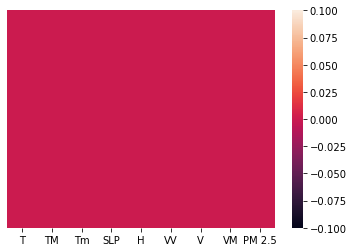Let us check outliers in our data using seaborn boxplot.

In :
# To check outliers
import matplotlib.pyplot as plt

a4_dims = (11.7, 8.27)
fig, ax = plt.subplots(figsize=a4_dims)
g = sns.boxplot(data=combine_data,linewidth=2.5,ax=ax)
g.set_yscale("log")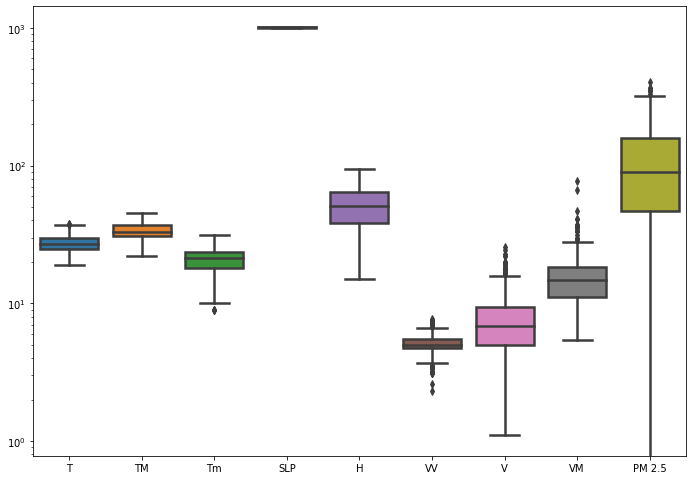From the plot, we can see that there are few outliers present in column Tm, W, V, VM and PM 2.5.

We can also do a searborn pairplot multivariate analysis. Using multivariate analysis, we can find out relation between any two variables. Since plot is so big, i am skipping the pairplot, but the command to draw pairplots are shown below.

In :
sns.pairplot(combine_data)


We can also check the corelation between dependent and independent features using dataframe.corr() function. The correlation can be plotted using 'pearson', 'kendall, or 'spearman'. By default corr() function runs 'pearson'.

In :
combine_data.corr()

Out:
T TM Tm SLP H VV V VM PM 2.5
T 1.000000 0.920752 0.786809 -0.516597 -0.477952 0.572818 0.160582 0.192456 -0.441826
TM 0.920752 1.000000 0.598095 -0.342692 -0.626362 0.560743 -0.002735 0.074952 -0.316378
Tm 0.786809 0.598095 1.000000 -0.735621 0.058105 0.296954 0.439133 0.377274 -0.591487
SLP -0.516597 -0.342692 -0.735621 1.000000 -0.250364 -0.187913 -0.610149 -0.506489 0.585046
H -0.477952 -0.626362 0.058105 -0.250364 1.000000 -0.565165 0.236208 0.145866 -0.153904
VV 0.572818 0.560743 0.296954 -0.187913 -0.565165 1.000000 0.034476 0.081239 -0.147582
V 0.160582 -0.002735 0.439133 -0.610149 0.236208 0.034476 1.000000 0.747435 -0.378281
VM 0.192456 0.074952 0.377274 -0.506489 0.145866 0.081239 0.747435 1.000000 -0.319558
PM 2.5 -0.441826 -0.316378 -0.591487 0.585046 -0.153904 -0.147582 -0.378281 -0.319558 1.000000

If we observe the above correlation table, it is clear that correlation between 'PM 2.5' feature and only SLP is positive. Corelation tells us if 'PM 2.5' increases what is the behaviour of other features. So if correlation is negative that means if one variable increases other variable decreases.

We can also Visualize Correlation Using Seaborn Heatmap.

In :
relation =combine_data.corr()
relation_index=relation.index

In :
relation_index

Out:
Index(['T', 'TM', 'Tm', 'SLP', 'H', 'VV', 'V', 'VM', 'PM 2.5'], dtype='object')
In :
sns.heatmap(combine_data[relation_index].corr(),annot=True)

Out:
<AxesSubplot:>Upto now, we have done only feature engineering. In next section, we will do feature selection.

## Feature Selection

In :
from sklearn.ensemble import RandomForestRegressor
from sklearn.model_selection import train_test_split
from sklearn.metrics import mean_squared_error as mse


Splitting the data into train and test data sets.

In :
X_train, X_test, y_train, y_test = train_test_split(
combine_data.iloc[:,:-1],
combine_data.iloc[:,-1],
test_size=0.3,
random_state=0)

In :
# size of train data set
X_train.shape

Out:
(450, 8)
In :
# size of test data set
X_test.shape

Out:
(193, 8)

Feature selection by ExtraTreesRegressor(model based). ExtraTreesRegressor helps us find the features which are most important.

In :
# Feature selection by ExtraTreesRegressor(model based)

from sklearn.ensemble import ExtraTreesRegressor
from sklearn.model_selection import train_test_split
from sklearn.metrics import accuracy_score as acc

In :
X_train, X_test, y_train, y_test = train_test_split(
combine_data.iloc[:,:-1],
combine_data.iloc[:,-1],
test_size=0.3,
random_state=0)

In :
reg= ExtraTreesRegressor()

In :
reg.fit(X_train,y_train)

Out:
ExtraTreesRegressor()
In [ ]:
Let us print the features importance.

In :
reg.feature_importances_

Out:
array([0.17525632, 0.09237557, 0.21175783, 0.22835392, 0.0863817 ,
0.05711284, 0.07977977, 0.06898204])
In :
feat_importances = pd.Series(reg.feature_importances_, index=X_train.columns)
feat_importances.nlargest(5).plot(kind='barh')
plt.show()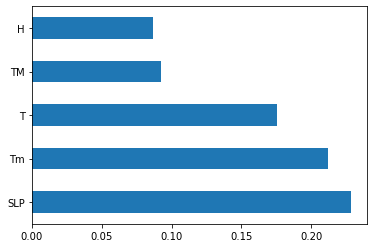Based on plot above, we can select the features which will be most important for our prediction model.

Before Train the data we need to do feature normalization because models such as decision trees are very sensitive to the scale of features.

## Decision Tree Model Training

In :
# Traning model with all features

from sklearn.model_selection import train_test_split
X_train, X_test, y_train, y_test = train_test_split(combine_data.iloc[:,:-1], combine_data.iloc[:,-1], test_size=0.3, random_state=0)

In :
X_train

Out:
T TM Tm SLP H VV V VM
334 28.9 36.0 15.0 1009.2 21.0 5.3 4.8 11.1
46 32.8 39.0 26.0 1006.6 41.0 5.6 7.0 77.8
246 30.3 37.0 24.2 1003.7 38.0 4.7 21.9 29.4
395 28.4 36.6 23.0 1003.1 63.0 4.7 10.7 18.3
516 26.9 31.0 22.9 1003.0 76.0 4.0 7.8 16.5
... ... ... ... ... ... ... ... ...
9 23.7 30.4 17.0 1015.8 46.0 5.1 5.2 14.8
359 33.6 40.0 25.0 1006.9 36.0 5.8 6.1 11.1
192 24.9 30.4 19.0 1008.9 57.0 4.8 4.6 9.4
629 26.1 29.0 22.4 1001.2 87.0 5.0 14.1 22.2
559 23.8 30.2 17.9 1010.6 55.0 4.5 3.7 7.6

450 rows × 8 columns

In :
X_test

Out:
T TM Tm SLP H VV V VM
637 28.4 33.5 20.9 1013.1 63.0 5.3 6.1 66.5
165 20.7 30.1 9.0 1010.5 35.0 4.5 4.6 14.8
467 26.7 33.5 21.0 1010.9 37.0 5.1 5.7 11.1
311 26.0 31.0 20.4 1011.5 63.0 4.8 3.9 9.4
432 26.4 30.9 22.6 1010.0 75.0 4.2 7.6 16.5
... ... ... ... ... ... ... ... ...
249 27.2 32.3 22.0 1003.7 55.0 4.8 20.0 29.4
89 29.7 34.0 22.6 1003.8 56.0 5.5 13.5 27.8
293 22.3 30.3 11.4 1012.6 37.0 5.1 7.2 20.6
441 27.1 33.0 20.0 1010.7 49.0 4.2 6.1 18.3
478 25.6 32.0 19.0 1012.1 59.0 3.9 6.1 11.1

193 rows × 8 columns

In :
from sklearn.tree import DecisionTreeRegressor


Let us creat a Decision tree regression model.

In :
reg_decision_model=DecisionTreeRegressor()

In :
# fit independent varaibles to the dependent variables
reg_decision_model.fit(X_train,y_train)

Out:
DecisionTreeRegressor()
In :
reg_decision_model.score(X_train,y_train)

Out:
1.0
In :
reg_decision_model.score(X_test,y_test)

Out:
0.05768194549539718

We got 100% score on training data.

On test data we got 5.7% score because we did not provide any tuning parameters while intializing the tree as a result of which algorithm split the training data till the leaf node. Due to which depth of tree increased and our model did the overfitting.

That's why we are getting high score on our training data and less score on test data.

So to solve this problem we would use hyper parameter tuning.

We can use GridSearch or RandomizedSearch for hyper parameters tuning.

## Decision Tree Model Evaluation

In :
prediction=reg_decision_model.predict(X_test)


Let us do a distribution plot between our label y and predicted y values.

In :
# checking difference between labled y and predicted y
sns.distplot(y_test-prediction)

/home/abhiphull/anaconda3/envs/condapy36/lib/python3.6/site-packages/seaborn/distributions.py:2557: FutureWarning: distplot is a deprecated function and will be removed in a future version. Please adapt your code to use either displot (a figure-level function with similar flexibility) or histplot (an axes-level function for histograms).
warnings.warn(msg, FutureWarning)

Out:
<AxesSubplot:xlabel='PM 2.5', ylabel='Density'>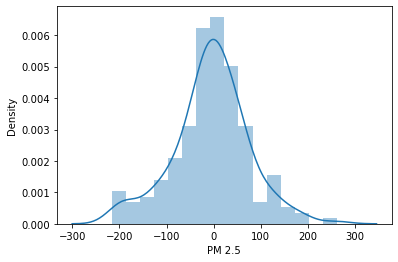We are getting nearly bell shape curve that means our model working good? No we can't make that conclusion. Good bell curve only tell us the range of predicted values are with in the same range as our original data range values are.

In [ ]:
checking predicted y and labeled y using a scatter plot.

In :
plt.scatter(y_test,prediction)

Out:
<matplotlib.collections.PathCollection at 0x7fa05aeb0320>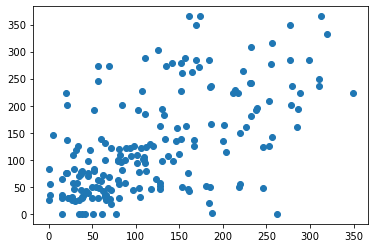## Hyper Parameter tuning

In :
# Hyper parameters range intialization for tuning

parameters={"splitter":["best","random"],
"max_depth" : [1,3,5,7,9,11,12],
"min_samples_leaf":[1,2,3,4,5,6,7,8,9,10],
"min_weight_fraction_leaf":[0.1,0.2,0.3,0.4,0.5,0.6,0.7,0.8,0.9],
"max_features":["auto","log2","sqrt",None],
"max_leaf_nodes":[None,10,20,30,40,50,60,70,80,90] }


Above we intialized hyperparmeters random range using Gridsearch to find the best parameters for our decision tree model.

In :
# calculating different regression metrics

from sklearn.model_selection import GridSearchCV

In :
tuning_model=GridSearchCV(reg_decision_model,param_grid=parameters,scoring='neg_mean_squared_error',cv=3,verbose=3)

In :
# function for calculating how much time take for hyperparameter tuning

def timer(start_time=None):
if not start_time:
start_time=datetime.now()
return start_time
elif start_time:
thour,temp_sec=divmod((datetime.now()-start_time).total_seconds(),3600)
tmin,tsec=divmod(temp_sec,60)
#print(thour,":",tmin,':',round(tsec,2))

In :
X=combine_data.iloc[:,:-1]

In :
y=combine_data.iloc[:,-1]

In :
%%capture
from datetime import datetime

start_time=timer(None)

tuning_model.fit(X,y)

timer(start_time)


Hyper parameter tuning took around 17 minues. It might vary depending upon your machine.

In :
# best hyperparameters
tuning_model.best_params_

Out:
{'max_depth': 5,
'max_features': 'auto',
'max_leaf_nodes': 40,
'min_samples_leaf': 2,
'min_weight_fraction_leaf': 0.1,
'splitter': 'random'}
In :
# best model score
tuning_model.best_score_

Out:
-3786.5642998048047

## Training Decision Tree With Best Hyperparameters

In :
tuned_hyper_model= DecisionTreeRegressor(max_depth=5,max_features='auto',max_leaf_nodes=50,min_samples_leaf=2,min_weight_fraction_leaf=0.1,splitter='random')

In :
# fitting model

tuned_hyper_model.fit(X_train,y_train)

Out:
DecisionTreeRegressor(max_depth=5, max_features='auto', max_leaf_nodes=50,
min_samples_leaf=2, min_weight_fraction_leaf=0.1,
splitter='random')
In :
# prediction

tuned_pred=tuned_hyper_model.predict(X_test)

In :
plt.scatter(y_test,tuned_pred)

Out:
<matplotlib.collections.PathCollection at 0x7fa05ac52c50>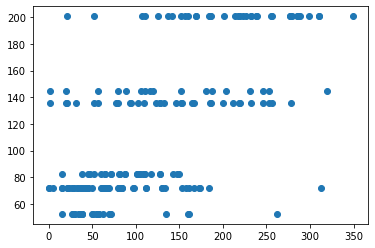Ok the above scatter plot looks lot better.

Let us compare now Error rate of our model with hyper tuning of paramerters to our original model which is without the tuning of parameters.

In :
# With hyperparameter tuned

from sklearn import metrics

print('MAE:', metrics.mean_absolute_error(y_test,tuned_pred))
print('MSE:', metrics.mean_squared_error(y_test, tuned_pred))
print('RMSE:', np.sqrt(metrics.mean_squared_error(y_test, tuned_pred)))

MAE: 48.814175526595086
MSE: 4155.120637935324
RMSE: 64.46022523956401

In :
# without hyperparameter tuning

from sklearn import metrics

print('MAE:', metrics.mean_absolute_error(y_test,prediction))
print('MSE:', metrics.mean_squared_error(y_test, prediction))
print('RMSE:', np.sqrt(metrics.mean_squared_error(y_test, prediction)))

MAE: 59.15023747989637
MSE: 6426.809819039633
RMSE: 80.16738625550688


## Conclusion

If you observe the above metrics for both the models, We got good metric values(MSE 4155) with hyperparameter tuning model compare to model without hyper parameter tuning.